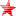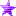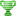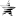# Expression syntax (CNS) (Read 961 times)

Started by Just No Point, October 07, 2015, 04:17:30 am
Share this topic:

#### Just No Point##### Expression syntax (CNS)
#1  October 07, 2015, 04:17:30 am
••Basically, any normal arithmetic expression is allowable. In addition, since the relational operators (>, <=, etc.) are viewed as returning ints, it is possible to operate on their return values, giving some unusual-looking expressions like

Code:
`1.0 = (2 = (1 > 0) + !(0 < 1))`
The 1 > 0 term evaluates to 1, and the 0 < 1 term evaluates to 0. Hence !(0 < 1) evaluates to 1, so the expression simplifies to

Code:
`1.0 = (2 = 1 + 1)`
Since 2 = 1 + 1, the term in parentheses evaluates to 1, so the expression further simplifies (after type conversion) to

Code:
`1.0 = 1.0`
which evaluates to 1 (int), since the equality holds.

A notable restriction in expression syntax is that interval operators are only allowed to appear on the rightmost side of an expression. If part of an expression is enclosed in parentheses, then that part is considered a subexpression, and an interval is allowed to appear on the right side of that subexpression. So the following is a well-formed expression, which evaluates to 0:

Code:
`(1 = [0,2]) = (0,1)`
But the following is not well-formed:

Code:
`1 = [0,2] = (0,1)`
In addition, no operator symbols other than = or != may appear before an interval. So an expression like 5 > [0,2], or 4 + [1,4), is not allowed.

In comma-separated parameter lists, such as the arguments to some function-type triggers or parameters to state controllers, each expression in the list is considered a separate subexpression, and therefore intervals may appear at the end of those subexpressions.